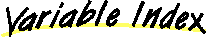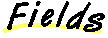`All Packages  Class Hierarchy  This Package  Previous  Next  Index`

# Class juice.operator.MatrixRep

```java.lang.Object
|
+----juice.operator.MatrixRep
```

public class MatrixRep
extends Object
A MatrixRep is a 2D array of floats which represent a specific linear operator. The representation is with respect to two bases, one of the operator's domain, and the other of the operator's range.

##AddomainBasisrangeBasis

##MatrixRep(LinearOperator)MatrixRep(LinearOperator, Basis, Basis)

##getDomainBasis()getOperator()getRangeBasis()getValue(int, int)toStringMatlab()
Converts the matrix into a form readable by Matlab.

Example:
println("A = " + myMatrixRep.toStringMatlab);
piping the output of this example to a file mymat.m will allow you to type "mymat" at the Matlab prompt to define the variable mymat to the matrix myMatrixRep.

##A
``` protected LinearOperator A
```domainBasis
``` protected Basis domainBasis
```rangeBasis
``` protected Basis rangeBasis
```d
``` protected float d[][]
```

##MatrixRep
``` public MatrixRep(LinearOperator A)
```MatrixRep
``` public MatrixRep(LinearOperator A,
Basis domainBasis,
Basis rangeBasis)
```

##getDomainBasis
``` public Basis getDomainBasis()
```getRangeBasis
``` public Basis getRangeBasis()
```getOperator
``` public LinearOperator getOperator()
```getValue
``` public float getValue(int i,
int j)
```toStringMatlab
``` public String toStringMatlab()
```
Converts the matrix into a form readable by Matlab.

Example:
println("A = " + myMatrixRep.toStringMatlab);
piping the output of this example to a file mymat.m will allow you to type "mymat" at the Matlab prompt to define the variable mymat to the matrix myMatrixRep.

`All Packages  Class Hierarchy  This Package  Previous  Next  Index`